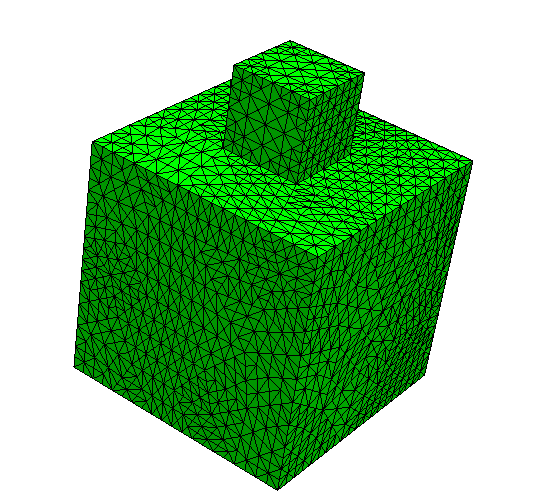# Adaptive refinement in 3D - Segmentation FaultI try to refine this mesh adaptively, but it dies at a.Assemble() after 2 refinements, directly forcing the program to an end, even without an error message. But if I refine it uniformly, it keeps working even after 4 refinements. Does anyone have an idea about what is going on here? The following is part of my code.

Update: I rerun the program on a Linux machine, and it shows `Segmentation fault (core dumped)`

``````# solve for the free dofs
def SolveBVP():
# assemble the bilinear and the linear form
a.Assemble()
l.Assemble()
# set up boundary conditions
if lam > 0:
if has_dirichlet:
uh.Set(mesh.BoundaryCF(bc["d"]), BND)
elif has_dirichlet or has_robin:
new_dirichlet_dict = {**bc["d"], **bc["r"]}
uh.Set(mesh.BoundaryCF(new_dirichlet_dict), BND)

# solve the linear system
#c.Update()
#solvers.BVP(bf=a, lf=l, gf=uh, pre=c)

r = l.vec - a.mat * uh.vec
inv = CGSolver(a.mat, c.mat)
uh.vec.data += inv * r

def CalcError():
# interpolate finite element flux into H(div) space:
gf_flux.Set (flux)

err = 1/d*(flux-gf_flux)*(flux-gf_flux)
eta2 = Integrate (err, mesh, VOL, element_wise=True)
eta2 = eta2.NumPy()
# Integrate ( *dx(element_boundary=True), mesh, VOL, element_wise=True)

max_eta2 = max(eta2)
sum_eta2 = sum(eta2)
errs.append((fes.ndof, sqrt(sum_eta2)))

# if we want to refine the mesh adaptively,
# we label the elements in need of refinements here
# *** MARK step
# Mark cells for refinement for which eta > frac eta_max for frac = .95, .90, ...;
# choose frac so that marked elements account for a given part of total error

frac = .95
delfrac = .05
part = .5
marked = zeros(mesh.ne, dtype=bool) # marked starts as False for all elements
sum_marked_eta2 = 0. # sum over marked elements of squared error indicators

while sum_marked_eta2 < part*sum_eta2:
new_marked = (~marked) & (eta2 > frac*max_eta2)
sum_marked_eta2 += sum(eta2[new_marked])
marked += new_marked
frac -= delfrac

for el in mesh.Elements():
mesh.SetRefinementFlag(el, marked[el.nr])

return sqrt(sum_eta2)

SolveBVP()
while CalcError() > tol and it < max_it:
mesh.Refine()
SolveBVP()
it += 1
``````

Attached is the information given by the debugger:

[Switching to Thread 0x7ffb1cd3a640 (LWP 2458752)]
0x00007ffdd459a058 in std::_Function_handler<void (ngcore::TaskInfo&), ngcore::ParallelFor<unsigned long, ngla::SparseMatrixTM::CreateTransposeTM(std::function<std::shared_ptr<ngla::SparseMatrixTM > (ngcore::Array<int, unsigned long> const&, int)> const&) const::{lambda(int)#2}>(ngcore::T_Range, ngla::SparseMatrixTM::CreateTransposeTM(std::function<std::shared_ptr<ngla::SparseMatrixTM > (ngcore::Array<int, unsigned long> const&, int)> const&) const::{lambda(int)#2}, int, ngcore::TotalCosts)::{lambda(ngcore::TaskInfo&)#1}>::_M_invoke(std::_Any_data const&, ngcore::TaskInfo&) () from /usr/lib/python3.10/dist-packages/ngsolve/…/…/…/netgen/libngla.so
#0 0x00007ffdd459a058 in std::_Function_handler<void (ngcore::TaskInfo&), ngcore::ParallelFor<unsigned long, ngla::SparseMatrixTM::CreateTransposeTM(std::function<std::shared_ptr<ngla::SparseMatrixTM > (ngcore::Array<int, unsigned long> const&, int)> const&) const::{lambda(int)#2}>(ngcore::T_Range, ngla::SparseMatrixTM::CreateTransposeTM(std::function<std::shared_ptr<ngla::SparseMatrixTM > (ngcore::Array<int, unsigned long> const&, int)> const&) const::{lambda(int)#2}, int, ngcore::TotalCosts)::{lambda(ngcore::TaskInfo&)#1}>::_M_invoke(std::_Any_data const&, ngcore::TaskInfo&) () from /usr/lib/python3.10/dist-packages/ngsolve/…/…/…/netgen/libngla.so
from /usr/lib/python3.10/dist-packages/netgen/…/…/…/netgen/libngcore.so
#2 0x00007ffff60dc253 in ?? () from /lib/x86_64-linux-gnu/libstdc++.so.6
#4 0x00007ffff7d26a40 in clone3 () at …/sysdeps/unix/sysv/linux/x86_64/clone3.S:81

difficult to say without running myself, send a (minimal) complete example reproducing the problem

Joachim

1 Like

Thanks for your reply! I played around with it a little bit and found that it works if I directly solve it instead of using a preconditioner. Do you have any idea what is happening here?

difficult to say without running myself, send a (minimal) complete example reproducing the problem

Joachim

bug.py (9.2 KB)

Thanks! Please see attached. If you use direct solver instead (comment Line 110, 129-131, uncomment Line 134), or you set is_adaptive = False (Line 231), you can run it without any problem.